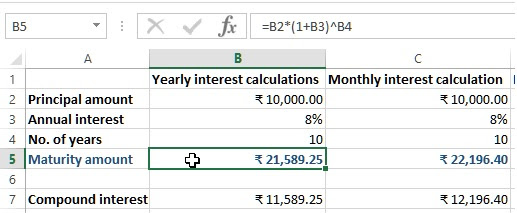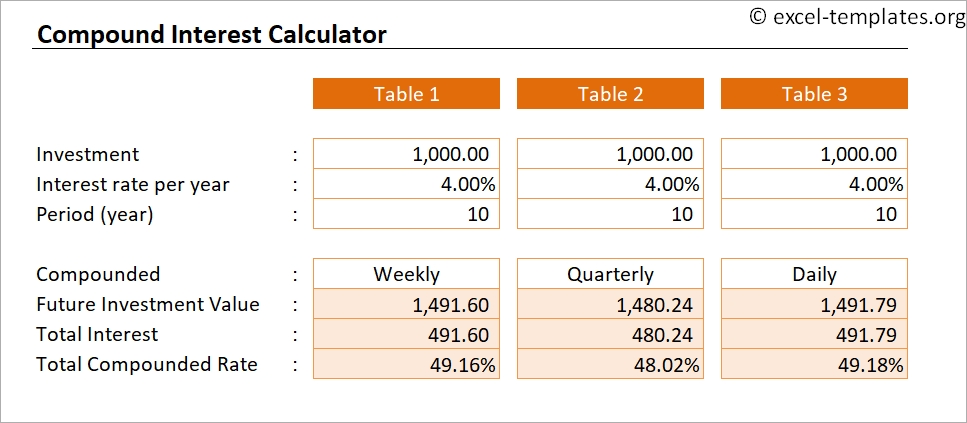Compound Interest Calculator is a ready-to-use excel template that helps to calculate compound interest with multiple compounding periods.

In addition to that, the template also provides a complete schedule of payments and interests accumulating each payment period.

## What is Compound Interest?

Compound interest is the method of calculating interest any given amount assuming that the interest earned each period is added to the principal. Hence, you receive not only interest on your principal amount but also the added interest each year.

While calculating compound interest the compounding periods matter the most and have a significant difference. For example, the amount of 10% compound interest compounded annually will be lower than 5% compound interest compounded semi-annually over the same time period.

### Formula To Calculate Compound Interest

Compound Interest (A) = P [(1 + i)n – 1]

Where: P = Principal Amount, i = interest rate, n = compounding periods.

## Compound Interest Calculator Excel Template

To simplify the process, we have created a simple and easy Compound Interest Calculator Excel Template with predefined formulas.

Just enter a few data and the template will calculate the compound interest for a particular investment. Additionally, the template also provides a schedule of payments and accumulated interests in each period.

Additionally, you can also download other financial analysis templates like NPV and XIRR Calculator, CAGR Calculator, ROCE Calculator, Income Tax Calculator FY 2018-19 and Loan Amortization Template from our website.

Let us understand the content of the templates in detail and how to use it.

## Contents of Compound Interest Calculator Excel Template

This template consists of 3 sheets: Basic Calculator, Advanced Calculator, and Cumulative Interest & Payment Schedule.### Basic Calculator

The basic calculator consists of 2 sections: Input and Output. Those cells having light-blue color is the input section and cells with dark blue are the output section.

The input section consists of the following heads:Principal Amount
Annual Interests Rate
Compounding Frequency
Years of Growth

The Output section is auto-populated based on the above-entered data. It consists of the following heads:

NPER
Rate: Rate per payment period.
Future Value: Future value is derived using the FV Function in Excel
Total Payments
Total Interest

Applying the compound interest formula the template calculates everything.

This sheet also consists of 2 sections: Input and Cumulative Income Report.

The input section is the same as the above. Additionally, it consists of a start date that will help to make the cumulative interest & payment schedule.

Compounding frequency and deposit frequency both have a drop-down list. Select the desired option fro the list where:

## Compound Interest Calculator Daily Formula

1 = Annually
2 = Semi-Annually
4 = Quarterly
6 = Bi-Monthly
12 = Monthly
24 = Semi-Monthly
26 = Bi-Weekly
52 = Weekly
365 = Daily

The payment frequency doesn’t have the “365” option in the dropdown of periods as it is not excepted daily basis.

## Compound Interest Calculator

Cumulative Income Report consists of the following heads:

Rate/Period: Rate per payment period.Total Payments: Principal Amount + Total Additional Payments.

Total Interest: Future Value – Total Payments.

### Cumulative Interest and Payment Schedule

The template creates a payment and interest schedule based on the data input in the Advanced Compound Interest Calculator. No entry is to be made on this sheet. It is auto-populated.

## How To Calculate Compound Interest

The Schedule sheet consists of the following columns:

## Investment Calculator Compound Interest

Sr. No: Serial Number of the payments. O is for the principal amount.

Date: Considering the start date and payment schedule it fetches the dates.

Net Payment: Principal amount + Interim Payment + Interest Amount.

Interest: The template uses the rates of Interest depending on compounding periods.

Cumulative Interest: Previous Interest + Current Interest.

## Compound Interest Equation Calculator

Balance: This column has the final balance depending on the tenure. Furthermore, It displays the value at the end of each period as well as at the completion of the period.

Thus, this template can be helpful to individuals planning to invest in fixed deposits in banks and for loan purposes.

We thank our readers for liking, sharing and following us on different social media platforms.If you have any queries please share in the comment section below. We will be more than happy to assist you.